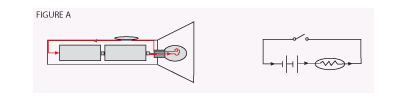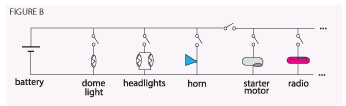Thursday, 09 May 2013 12:00

## Basic Electricity, Part 2

Written by   SCMHR
Rate this item
(1 Vote)

### Ohm's Law

Ohm's Law is the expression of voltage, current and resistance in an electrical circuit. First, we must define these parameters:

Voltage is defined as electrical force or pressure. A battery has voltage when it is electrically charged, and ready to do electrical work. This is similar to water pressure in a water system. When the water tower is full, it has pressure (voltage) and is ready to do work when the faucet is open.

Current is defined as the rate of flow of electrons past a given point in an electrical circuit. This is comparable with how fast the water comes out of the faucet when the faucet is open in a water system.
Resistance is defined as the resistance to a flow of electrons in an electrical circuit. This is comparable to the size of the pipe, or how far the faucet is opened in a water system.

Ohm's Law stated mathematically is as follows: Voltage equals current times resistance (voltage = current x resistance).

The mathematical symbols used in Ohm's Law are:

Voltage = E
Current = I
Resistance = R

Then, when we know any two parameters, we can mathematically calculate the third.

E = I x R, I = E/R, R = E/I

Where "x" means multiply, and "/"
means divide.

### Electrical Components

Let us define some electrical components:

Battery: or wall outlet: source of voltage (direct current or alternating current).
Conductor: device along which electrons can flow (usually a wire).
Resistor: device that presents resistance to a flow of electrons (usually made of carbon).
Insulator: device that presents virtual total opposition to a flow of electrons (the ultimate resistor).
Fuse: a device which protects an electrical circuit from an overload condition.
Circuit Breaker: same as a fuse, only more convenient (no need to replace – just reset).
Transformer: device to step-up (raise), or step-down (lower) alternating current.
Rectifier: device that changes alternating current to direct current (be careful! It does not work the other way – D.C. to A.C.).
Filter: device to smooth out alternating current.
Inductor: device to smooth out direct current.
Rheostat: variable resistor (almost any electrical component with a knob) as in timing and intensity control on an epilator, volume control on a radio, et cetera.

###A Simple Circuit

The circuit, or schematic, shown on the next page (FIGURE A), demonstrates a simple series circuit. Because it appears as a battery, it is a direct current (or D.C.) circuit. When the switch (shown in the on or off position) is closed, the negative electrons will leave the negative pole (or terminal) of the battery, flow along the conductor, and be attracted by the law of charges to the positive pole. On the way the electrons will have to pass through the lightbulb. Here they will meet resistance, as the filament of the lightbulb is made of a material called tungsten, which is not a very good conductor of electricity. As the electrons force their way through the resistance of the lightbulb, the tungsten filament becomes white hot and gives off light.

### Series Circuit

If two bulbs were connected in a series (one after the other) and one bulb burned out, neither bulb would light because the circuit would become "open." For example, remember the old-fashioned Christmas tree lights? When one bulb burned out, they all went out; and it took a long time to find the defective bulb.

### Parallel Circuit

This is similar to the series circuit, except now the electrons have two paths to choose; they may travel through either the first or second lightbulb (FIGURE B). If both lightbulbs are the same, as in the headlights of your car, then half will go through the other bulb (FIGURE B). The advantage of this type of circuit is that if one bulb burns out, the other will still give light. An alternating current equivalent of this type of circuit is a modern Christmas tree light set. In this case, there are many bulbs connected in parallel, and when one bulb burns out, the rest stay lit.

### Power

Electrical power is defined as the ability to do electrical work. It is mathematically determined by multiplying voltage (E) times current (I). Voltage without current, and conversely, current without voltage cannot accomplish much meaningful electrical work. The proper term for electrical power is watt. When we buy lightbulbs, we buy them according to their watts; the more watts, the brighter the light, and the larger the electrical bill.

###Power and Time

The concept of power and time is critical in electrolysis, especially in regards to galvanic epilation and short wave epilators operating on automatic timing. When we receive our electric bill, we are billed from the power company for a quantity of kilowatt-hours at a rate of so many cents per kilowatt-hour. The prefix kilo means 1,000; therefore, a kilowatt means 1,000 watts. If your power company charges you seven cents per kilowatt hour, then it follows that if you burn a 1,000 watt lightbulb for one hour (1,000 watts x one hour = one kilowatt hour) it would cost you seven cents. Similarly, if you would only burn a 500-watt light bulb for one hour, you could cut your bill in half.

### Joules

In electrolysis, we do not introduce very much electrical power into the follicle, and we do not have the current flowing for very long periods of time. Fortunately, there is a little cousin of the kilowatt-hour that serves our purposes in electrolysis. The joule is defined as a watt-second. In other words, it is a tiny kilowatt-hour. Most shortwave epilators have a maximum power output capability of approximately 10 watts, or less. The automatic timing circuits of most shortwave epilators are capable of maximum timing duration of one second or less. Therefore, one can readily see that if an epilator had a maximum power output capability of 10 watts and a maximum automatic timing cycle of one second, then that epilator would have the potential to deliver a maximum of 10 joules to the follicle in automatic mode. From this, one can readily see that the amount of energy delivered to the follicle in the form of heat, with a shortwave epilator operating in the automatic mode, is the product (multiplication) of timing and intensity. The important point here is that timing and intensity both play equally important roles in successful epilation.

You may earn continuing education credit for this two-part article by passing a short test on the material and paying a small administrative fee. For more information, please contact the Society for Clinical and Medical Hair Removal's home office at
608-443-2470 or This email address is being protected from spambots. You need JavaScript enabled to view it..